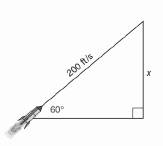Chapter 11.CR, Problem 22CRElementary Geometry For College St...

7th Edition
Alexander + 2 others
ISBN: 9781337614085

Solutions

Chapter
SectionElementary Geometry For College St...

7th Edition
Alexander + 2 others
ISBN: 9781337614085
Textbook Problem

In Review exercises 21 to 30. Use the drawings. Where provided, to solve each problem. Angle measures should be found to the nearest degree; lengths should be found to the nearest tenth of a unit.A rocket is shot into the air at angle of 60 ° If it is traveling at 200 ft per second, how high in the air is it after 5 seconds? (Ignoring gravity, assume that the path of the rocket is a straight line.)To determine

To find:

The height of the rocket in air after 5 seconds of its shot at an angle of 60° and it is travelling at 200 ft per second.

Explanation

Procedure used:

In any right triangle ABC with mA=θ we have the following ratios.

sinθ=OppositeHypotenuse

Calculation:

Given:

Speed of the rocket =200 ft per second.

Angle of projection (elevation) =60°

The height of the rocket in air after 5 seconds =x.

Let us structure the given figure as follows as a right triangle.

First let us find the distance travelled by the rocket in 5 second.

Distance travelled = Speed × Time

Distance travelled by the rocket

=200×5

=1000 ft

Still sussing out bartleby?

Check out a sample textbook solution.

See a sample solution

The Solution to Your Study Problems

Bartleby provides explanations to thousands of textbook problems written by our experts, many with advanced degrees!

Get Started

17. Let and Does ? Find . Does Find and simplify.

Mathematical Applications for the Management, Life, and Social Sciences

In Exercises 516, evaluate the given quantity. log1616

Finite Mathematics and Applied Calculus (MindTap Course List)

Evaluate the indefinite integral. cos(1+5t)dt

Single Variable Calculus: Early Transcendentals, Volume I

Perform the indicated operation for the following. 22.

Contemporary Mathematics for Business & Consumers

ex(ex)2 = a) ex3 b) e3x c) 3ex d) e4x

Study Guide for Stewart's Single Variable Calculus: Early Transcendentals, 8th